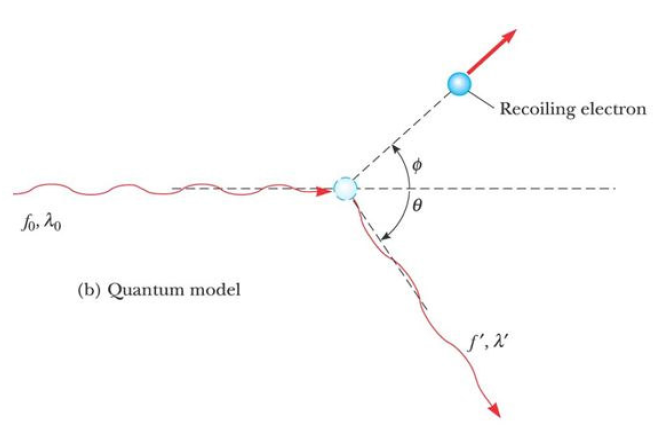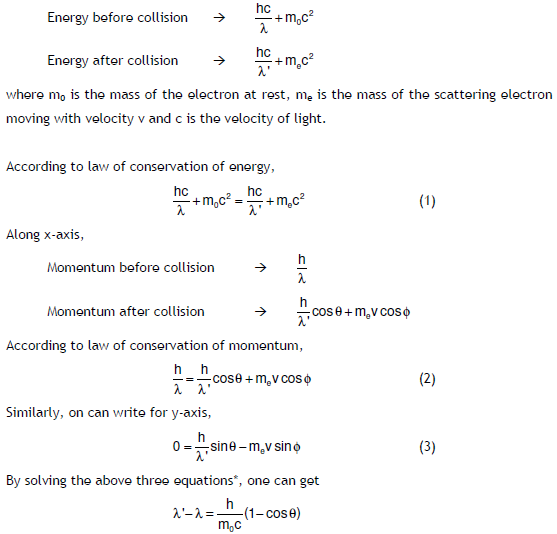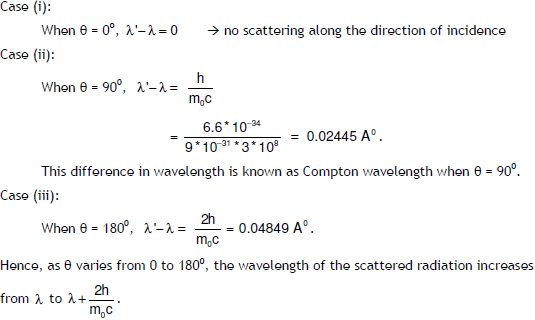Compton Effect: Compton (1923) measured intensity of scattered X-rays from solid target, as function of wavelength for different angles. He got Nobel prize for that in 1927.
When a beam of monochromatic radiation such as X-rays, -ray, etc., of high frequency is allowed to fall on a fine scatterer, the beam is scattered into two components namely

•  one component having the same frequency or wavelength as that of the incident radiation, so called unmodified radiation and
•  the other component having lower frequency or higher wavelength compared to incident radiation, so called modified radiation.Let us consider the collision which occurs between the photon (having initially the energy hv =hc/λ) and electron. The electron is free and is at rest before collision with the incident photon. During collision, a part of energy is given to the electron, which in turn increases the K.E. of the electron and hence it recoils at an angle of 9 as shown in figure. The scattered photon moves with an energy hv' = hc/λ' (< hn ) at an angle : with respect to the original direction. Obviously, one can use law of conservation of energy and momentum for such a system. For that, we have to know the momentum and energy values before and after collision.which is the change (or shift) in wavelength. It is clear that the wavelength shift is independent of the wavelength of the incident radiation λ as well as the nature of the scatterer and it is found to depend on the angle of scattering :.